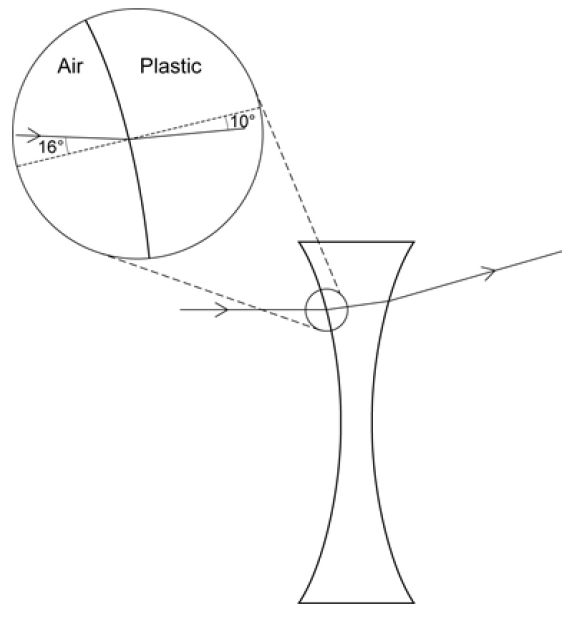# Lenses – Question 1

The diagram shows a ray of light passing through a diverging lens.(a) Use the information in the diagram to calculate the refractive index of the plastic used to make the lens.

Write down the equation you use, and then show clearly how you work out your answer.Refractive Index = ................................
(2 marks)

(b) The focal length of the lens is 5 cm. A student looking through the lens sees the image of a pin.

Complete the ray diagram below to show how the image of the pin is formed.(3 marks)

How did you do? Click to show the mark schemeFor part (b):From Q6 P3 AQA Specimen Paper (Higher)